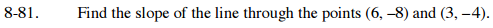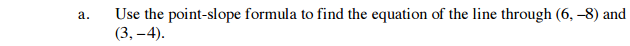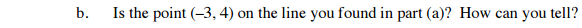### Home > CAAC > Chapter 8 > Lesson 8.2.4 > Problem8-81

8-81.yk = m(xh)
Where (h, k) is a point on the line and m is the slope.Substitute the coordinates into the equation to see if the point is on the line.

$y=-\frac{4}{3}x$